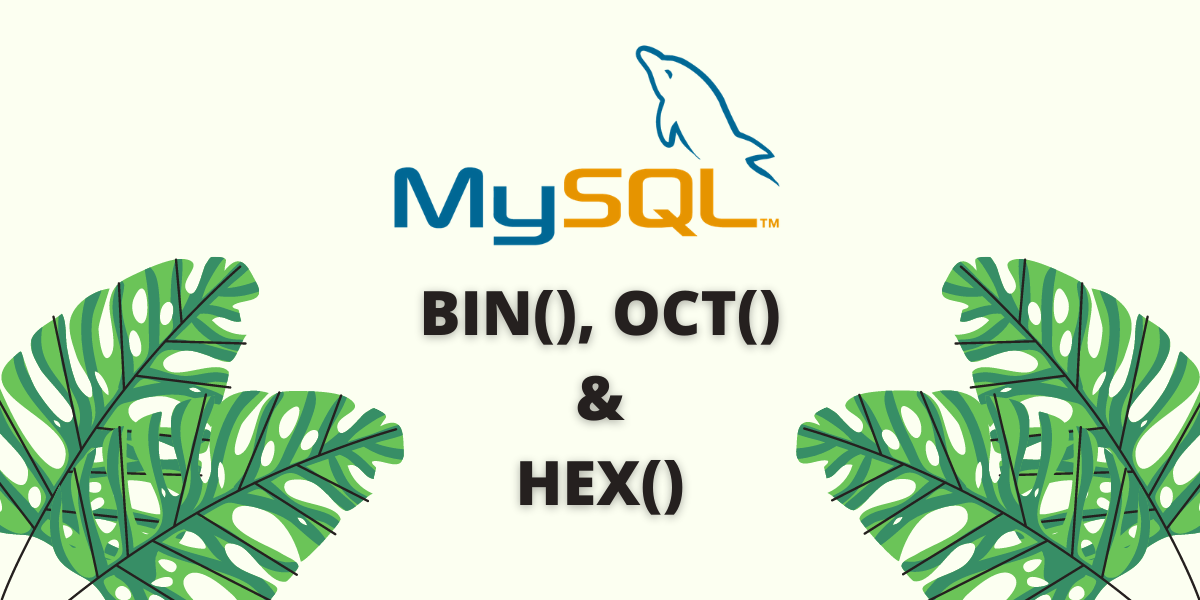# MySQL BIN(), OCT() and HEX() FunctionsIn this tutorial, we will learn about the MySQL `BIN()`, `OCT()` and `HEX()` functions. As you would have known by now, computers do not understand the language of humans. They only understand 0s and 1s. Numbers in the computer language often have a base or radix, such that all numbers present in that number system range from zero to (base-1). Let me elaborate more on this.

## Introduction

In computer language, there are four popular number systems –

• The decimal number system – The base is 10.
• The binary number system – The base is 2.
• The octal number system – The base is 8.
• The hexadecimal number system – The base is 16.

These numbers find a lot of applications in electronic and digital circuits which, long story short, form the basis of our modern-day computers. The decimal number system is the number system which we use in our everyday life. Let us explore the other number systems using the MySQL `BIN()`, `OCT()` and `HEX()` functions.

## MySQL BIN()

The MySQL `BIN()` function is used to convert a number (from the decimal number system) to its binary equivalent. The returned value is a string.

Following is a table that shows the binary representation of numbers from 0 to 8. Note that numbers greater than 8 also have binary equivalent values. This table is just for example purposes.

### Syntax of MySQL BIN()

```.wp-block-code {
border: 0;
}

.wp-block-code > span {
display: block;
overflow: auto;
}

.shcb-language {
border: 0;
clip: rect(1px, 1px, 1px, 1px);
-webkit-clip-path: inset(50%);
clip-path: inset(50%);
height: 1px;
margin: -1px;
overflow: hidden;
position: absolute;
width: 1px;
word-wrap: normal;
word-break: normal;
}

.hljs {
box-sizing: border-box;
}

.hljs.shcb-code-table {
display: table;
width: 100%;
}

.hljs.shcb-code-table > .shcb-loc {
color: inherit;
display: table-row;
width: 100%;
}

.hljs.shcb-code-table .shcb-loc > span {
display: table-cell;
}

.wp-block-code code.hljs:not(.shcb-wrap-lines) {
white-space: pre;
}

.wp-block-code code.hljs.shcb-wrap-lines {
white-space: pre-wrap;
}

.hljs.shcb-line-numbers {
border-spacing: 0;
counter-reset: line;
}

.hljs.shcb-line-numbers > .shcb-loc {
counter-increment: line;
}

.hljs.shcb-line-numbers .shcb-loc > span {
}

.hljs.shcb-line-numbers .shcb-loc::before {
border-right: 1px solid #ddd;
content: counter(line);
display: table-cell;
text-align: right;
-webkit-user-select: none;
-moz-user-select: none;
-ms-user-select: none;
user-select: none;
white-space: nowrap;
width: 1%;
}`BIN(number)`Code language: SQL (Structured Query Language) (sql)```

Where ‘number’ is a number whose binary representation is to be found out.

### Example of MySQL BIN()

Let us find the binary representation of the following numbers – 2, 15 and 22. The queries are –

``````SELECT BIN(2);
SELECT BIN(15);
SELECT BIN(22); ```Code language: SQL (Structured Query Language) (sql)```

And we get the output as follows.

## MySQL OCT()

The MySQL `OCT()` function is used to convert a number (from the decimal number system) to its octal equivalent. The returned value is a string.

Following is a table that shows the octal representation of numbers from 0 to 10. Note that numbers greater than 10 also have octal equivalent values. This table is just for example purposes.

### Syntax of MySQL OCT()

``OCT(number)`Code language: SQL (Structured Query Language) (sql)`

Where ‘number’ is a number whose octal representation is to be found out.

### Basic Example of MySQL OCT()

Let us find the octal representation of the following numbers – 2, 15, 9, 8 and 7. The queries are –

``````SELECT OCT(2);
SELECT OCT(15);
SELECT OCT(9);
SELECT OCT(8);
SELECT OCT(7);```Code language: SQL (Structured Query Language) (sql)```

And we get the output as follows.

## MySQL HEX()

The MySQL `HEX()` function is used to convert a number (from the decimal number system) to its hexadecimal equivalent. The returned value is a string.

Following is a table that shows the hexadecimal representation of numbers from 0 to 18. Note that numbers greater than 18 also have hexadecimal equivalent values. This table is just for example purposes.

### Syntax of MySQL HEX()

``HEX(number)`Code language: SQL (Structured Query Language) (sql)`

Where ‘number’ is a number whose hexadecimal representation is to be found out.

### Basic Example of MySQL HEX()

Let us find the hexadecimal representation of the following numbers – 7, 11, 17, 22 and 28. The queries are –

``````SELECT HEX(7);
SELECT HEX(11);
SELECT HEX(17);
SELECT HEX(22);
SELECT HEX(28);```Code language: SQL (Structured Query Language) (sql)```

And we get the output as follows.

## MySQL BIN(), OCT() and HEX() With Tables

Consider the below ‘Integers’ table. It has only one column – the Number column which stores an integer.

Using the `SELECT` Statement, aliases and the `BIN()`, `OCT()` and `HEX()` functions; let us find the binary, octal and hexadecimal equivalent values of the values in the Number column. The query is –
``SELECT Number, BIN(Number) AS BinaryValue, OCT(Number) AS OctalValue, HEX(Number) AS HexadecimalValue FROM Integers;`Code language: SQL (Structured Query Language) (sql)`